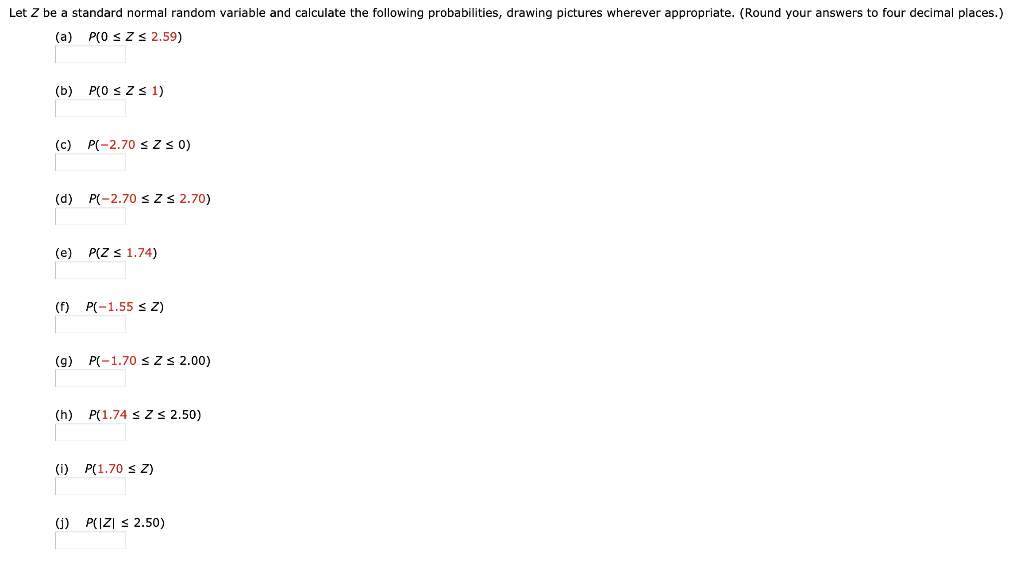Solved: Let Z Be A Standard Normal Random Variable And Calculate The Following Probabilities, Drawing Pictures Wherever Appropriate. (Round Your Answers To Four Decimal Places.) (a) P(0 Zs 2.59) (b) P

By |Let Z be a standard normal random variable and calculate the following probabilities, drawing pictures wherever appropriate. (Round your answers to four decimal places.) (a) P(0 Zs 2.59) (b) P(0 s Z s 1) (c) P-2.70 s Z s 0) (d) P-2.70 s Z s 2.70) P(Z 1.74) (e) P(-1.55 s ) (f) (g) P-1.70 s Z s 2.00) (h) P(1.74 s Z s 2.50) (i) P(1.70 s Z) (J) PCIZI2.50)